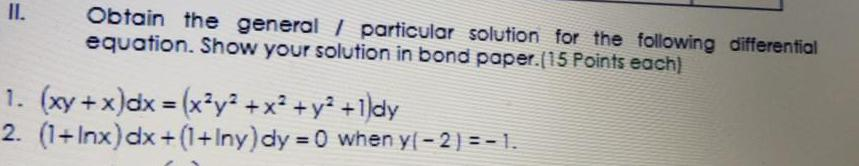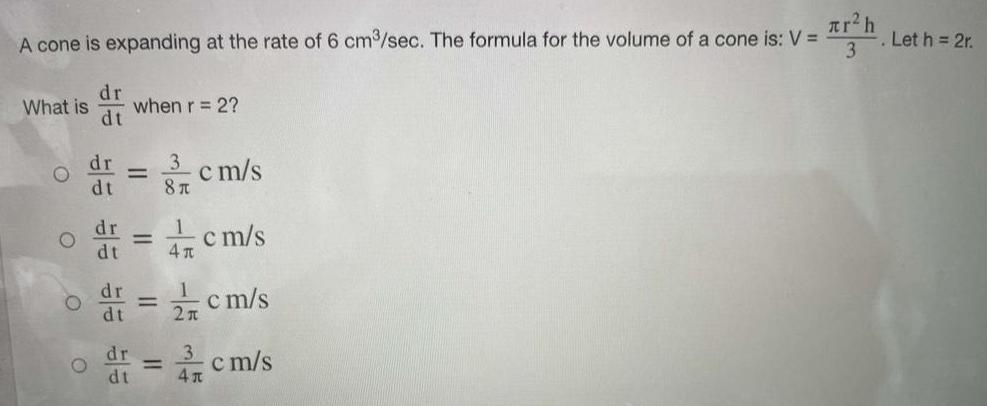# Differential equations Questions and AnswersMath
Differential equations
II. Obtain the general / particular solution for the following differential equation. Show your solution in bond paper.(15 Points each) 1. (xy + x) dx = (x³y² + x² + y² +1)dy 2. (1+Inx) dx+(1+Iny) dy =0 when y(-2) = -1.Math
Differential equations
A cone is expanding at the rate of 6 cm³/sec. The formula for the volume of a cone is: What is when r = 2?